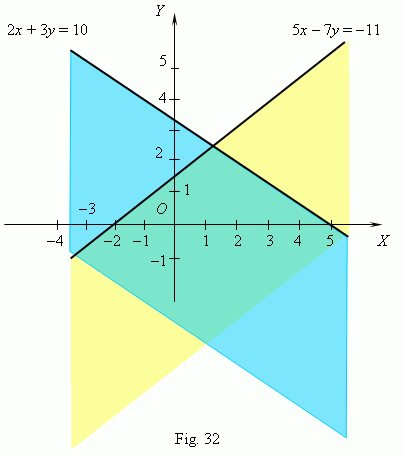# Graphical solving of inequalities

Approximate solution of inequalities. Graphical solving of
inequalities in one unknown. Graphical solving of systems
of simultaneous inequalities in two unknowns. Intersection
of solutions.

Graphical representation of functions permits to solve approximately inequalities in one unknown and systems of simultaneous inequalities in one and two unknowns. To solve an inequality in one unknown graphically, it is necessary to transfer all its terms to the same part, i.e. to reduce it to the shape:

f ( x ) > 0 ,

and to build a graph of the function  y = f ( x ) . After this, using the built graph, it is possible to find zeros of the function (see above ), which divide an x -axis into some intervals. Now on this base we’ll determine intervals of argument x , within which the function sign corresponds to the inequality. For instance, zeros of our function are: a and b ( Fig.30 ). Then from the graph it is obvious that intervals, within which f ( x ) > 0 are: x < a and x > b ( they are marked as bold arrows ). It’s clear, that a symbol  >  is conditional here; instead of it any other symbol can be: < ,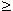,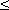.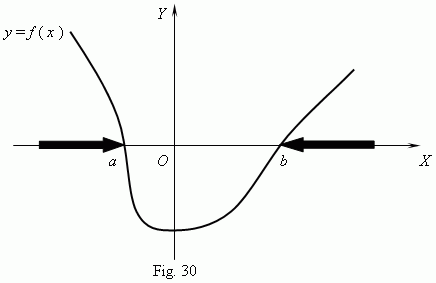To solve a system of simultaneous inequalities in one unknown graphically, it is necessary to transfer in each of them all terms to the same part, i.e. to reduce the inequalities to the shape: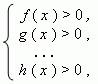and to build graphs of the functions  y = f ( x ), y = g ( x ) , ... , y = h ( x ). Each of these inequalities can be solved by graphical method, written above. After this it is necessary to find an intersection of all inequalities solutions, i.e. their common part.

E x a m p l e .  Solve graphically the system of simultaneous inequalities: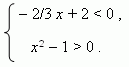S o l u t i o n .  Firstly we build graphs of the functions y = – 2 / 3 x + 2  and y = x 2 – 1 ( Fig.31 ):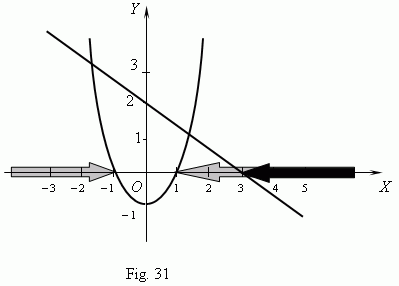The solution of the first inequality is the interval x > 3, marked on Fig.31 by a black arrow;  the second inequality has the solution, consisting of the two intervals: x < – 1 and x > 1, marked on  Fig.31  by grey arrows. We can see from this graph, that an intersection of these two solutions is the interval x > 3. This is the solution of the given system of simultaneous inequalities.

To solve a system of two simultaneous inequalities in two unknowns graphically, it is necessary:
1)   to transfer in each of them all terms to the same part,  i.e. to reduce the
inequalities to the shape: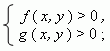2)   to build graphs of the functions, given by implicit formulas:  f ( x, y ) = 0  and g ( x, y ) = 0 ;

3)   each of these graphs divides a coordinate plane into two parts: in one of them the inequality is valid,
in the other it is not; to solve graphically each of these inequalities it is sufficient to check the inequality
validity at one arbitrary point in any part of the plane; if the inequality is valid at this point, then this   part

of a coordinate plane is the solution of the inequality, if not – an opposite part of the plane is the solution ;

4)   the solution of the given system of simultaneous inequalities is an intersection ( a common area ) of the parts
of a coordinate plane.

E x a m p l e .  Solve the system of simultaneous inequalities: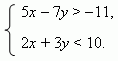S o l u t i o n .  Firstly we build graphs of the linear functions:  5 x – 7 y = – 11 and
2 x + 3 y = 10  ( Fig.32 ). For each of them we find a half-plane, in
which the given inequalities are valid. We know, that it is sufficient
to check a validity of the inequalities at any one point; here the easiest
point for check is an origin of coordinates O ( 0, 0 ). Substituting
its coordinates in our inequalities instead of x and y , we receive:
5 · 0 – 7 · 0 = 0 > – 11,  hence the lower half-plane (yellow) is the
solution for the first inequality;  2 · 0 + 3 · 0 = 0 < 10,  therefore the
second inequality has as a solution also the lower half-plane (blue).
An intersection of these half-planes (an aqua area) is the solution of
our system of simultaneous inequalities.